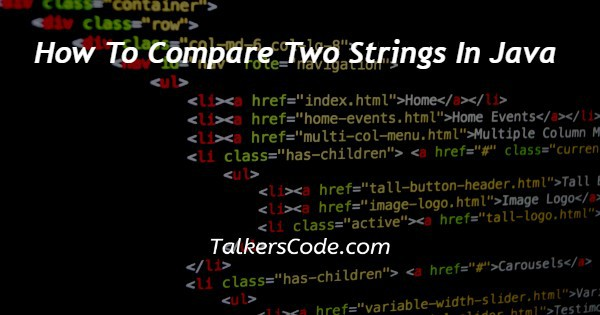# How To Compare Two Strings In Java

Last Updated : Jul 1, 2023In this article we will show you the solution of how to compare two strings in java, strings are sequences of characters. String objects in Java are immutable, that also means those who are constant and can't be changed once they are created.

### Here are five Java methods for comparing two Strings:

• Making use of a user-defined function: Create a function to try comparing values that meet the following criteria:
• If (string1 > string2), the result is positive.
• If both strings are lexicographically equal, i.e. (string1 == string2), it returns 0.
• If (string1 string2) is negative, it reverts a negative value.
• str1.charAt(i) - str2.charAt(i) (i)

### Using String.equalsIgnoreCase():

The String.equalsIgnoreCase() strategy compares two strings without regard to case (lower or upper).

This strategy evaluates to true is if argument isn't really null as well as the contents of the both Strings are the same, regardless of case, and false otherwise.

Syntax:

`str2.equalsIgnoreCase(str1);`

Here, str1 and str2 are both strings to be compared.

### Using Objects.equals():

The method Object.equals(Object a, Object b) evaluates to true if the arguments are equal and false otherwise.

As a result, true is returned when both arguments seem to be null, and false is returned if only one argument is null.

Alternatively, the equals() method of the first argument has been used to determine equality.

Syntax:

`equals public static boolean (Object a, Object b)`

In this case, a and b are indeed the string objects to be compared.

There are some distinctions between equals() and Java's "==" operator in terms of comparing objects for equality:

• As a method, the == operator differs from the equals() method in that it is an operator, not a method.
• For source comparison (address comparison), use == operators, and for content comparison, use the.equals() method.

In other words, == determines whether either objects point to the exact memory location, whereas.equals() compares the values in the objects.

In this case, two String objects, s1 and s2, are created. When the == operator is used to compare s1 and s2, the result seems to be false because they have multiple locations in memory.

Because equals is still only comparing the numbers in s1 and s2, the result is true.

## Step By Step Guide On How To Compare Two Strings In Java :-

```public class TalkersCode{
public static void main(String args[])
{
String string1 = new String("TalkersCode");
String string2 = new String("Talkers");
String string3 = new String("Code");
String string4 = new String("TalkersCode");
String string5 = new String("code");
System.out.println("Comparing " + string1 + " and " + string2
+ " : " + string1.equals(string2));
System.out.println("Comparing " + string3 + " and " + string4
+ " : " + string3.equals(string4));
System.out.println("Comparing " + string4 + " and " + string5
+ " : " + string4.equals(string5));
System.out.println("Comparing " + string1 + " and " + string4
+ " : " + string1.equals(string4));
}
}```
1. First, we must create a class called TalkersCode.
2. Next, we create a public static void main to begin the program's initialization.
3. Next, we create string objects to define value and compare values.
4. Finally, we equal the objects with true and false and exit the programme.

## Conclusion :-

A comparison using the compareTo() method compares two strings lexicographically and returns a value indicating whether first string is greater than, equal to, or less than second string.

I hope this article on how to compare two strings in java helps you and the steps and method mentioned above are easy to follow and implement.